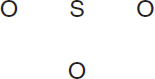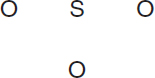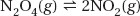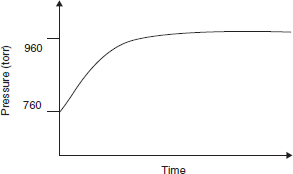# AP Chemistry Practice Test 29

### Test Information14 questions21 minutes

`Questions 1-4 refer to the following information.VesselABCGasBromomethaneDibromomethaneTetrabromomethaneFormulaCH3BrCH2Br2CBr4Molar mass95 g/mol174 g/mol332 g/molPressure0.2 atm0.4 atm0.2 atmTemperature600 °C500 °C500 °CNote that the gases are held in separate, identical, rigid vessels.`

1. Which sample has the lowest density?

2. The average kinetic energy

3. Which of these gases is expected to condense at the lowest pressure, assuming that the temperature is held constant?

4. Which attractive force is the major cause of condensation?

5.Use the arrangement of atoms suggested in the skeleton structure above to construct the Lewis structure for the SO3 molecule. Which of the following statements about this molecule is incorrect?

6.Use the arrangement of atoms suggested in the skeleton structure above to construct the Lewis structure for the sulfite ion. Be sure to minimize the formal charges. Which of the following statements is incorrect?

`Questions 7-9 refer to the following information.Pb(ClO4)2 + 2KCl → PbCl2(s) + 2KlO4A chemist mixes a dilute solution of lead perchlorate with a dilute solution of potassium chloride to precipitate lead(II) chloride (Ksp = 1.7 × 10-5).`

7. Which of the following is the net ionic equation for the experiment described above?

8. If equal volumes of 2.0 millimolar solutions are mixed, which of the following particulate views represents the experiment after the reactants are mixed thoroughly and the solids are given time to precipitate?

9. Within a factor of 10, what is the approximate molar solubility of PbCl2 in a solution of 0.17 M Pb2+ ions?

`Questions 10-14 refer to the following information.N2O4(g) decomposes into NO2(g) according to the equation above. A pure sample of N2O4(g) is placed into a rigid, evacuated 0.500 L container. The initial pressure of the N2O4(g) is 760 atm. The temperature is held constant until the N2O4(g) reaches equilibrium with its decomposition products. The figure below shows how the pressure of the system changes while reaching equilibrium.`

10. Which is a correct description of the partial pressures of N2O4 and NO2?

11. The pressure versus time curve can be used to evaluate

12. What will the final pressure be if this reaction goes to completion? Based on the data, does this reaction go to completion?

13. What is the appropriate combination of numbers for calculating Kp?

14. Which diagram is an appropriate description of the system if more NO2(g) is rapidly injected, at time = 0, into the container after equilibrium is established?